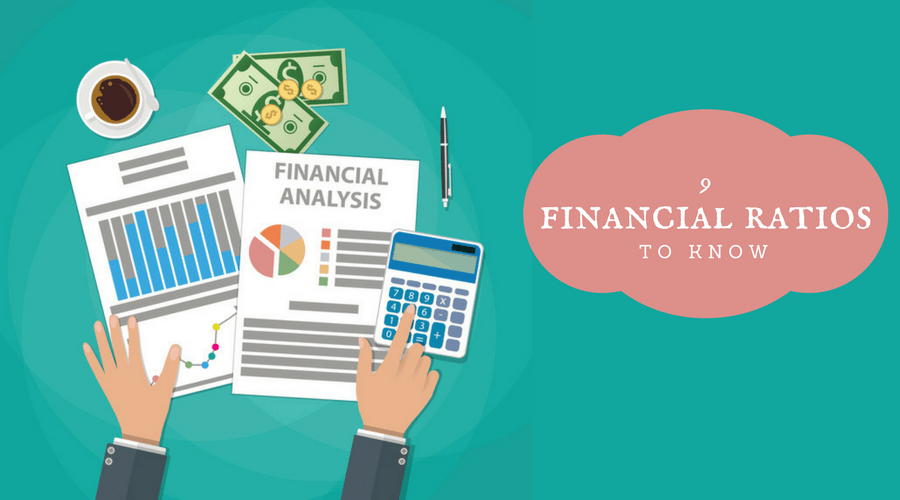### Financial Ratios

Vivianna De La Torre
July 6, 2022Key performance indicators are useful when understanding the financial position of a business to determine whether one should invest in a business and for business owners who want to keep track of the company’s performance. There are ratios to determine the liquidity, solvency, and profitability of a business. In this blog, I will go ahead and explain what these financial ratios are and how to calculate them. These ratios can be applied to one's own business, to analyze the financial position of another company, or to conduct an industry analysis of two or more companies in the same industry. All the numbers needed to calculate the KPIs can be found on a company’s financial statements provided in their 10ks.

Liquidity Ratios

Liquidity is the amount of liquid assets available to a company, cash being the most liquid asset. High liquidity indicates that a company can meet short-term obligations without having to take out loans. Some popular liquidity ratios include the current ratio, current cash debt coverage ratio, and inventory turnover ratio. There are more liquidity ratios, however, I will only be going over these three. The current ratio is calculated by dividing the current assets by the current liabilities. This ratio indicates the company’s ability to pay short-term debts. Generally, a current ratio above 1 is good, but a current ratio of 2 is more desirable. It is important to note that a good current ratio varies by industry. For example, for the retail industry, the average current ratio is 1.5. The current cash debt coverage ratio is calculated by dividing cash provided by operations by the average current liabilities. The current cash debt coverage ratio indicates the ability of a company to pay its current liabilities from its operations. A higher ratio indicates a better liquidity position, and an ideal ratio would be 1:1 because it demonstrates that the business is able to pay all of its current liabilities from the cash flow of its operations. The inventory turnover ratio is calculated by dividing the cost of goods sold by the average inventory. The inventory turnover ratio determines how many times a company has sold and replaced inventory within a given period of time. A high inventory turnover is ideal.

Solvency Ratios

Solvency is defined as the ability of the company to meet their long-term obligations. The ratios that I will be touching on are the times interest earned ratio, debt to equity ratio, and the free cash flow. The times interest earned ratio is calculated by dividing the operating income by the interest expense. This ratio demonstrates the ability to meet interest expense and income taxes. A higher times interest earned ratio is considered more favorable because it appears less risky to investors and creditors in terms of solvency. The debt to equity ratio is calculated by dividing the total debt by the total of all common equity. The debt to equity ratio is the relationship of debt to the company’s equity. A good debt to equity ratio varies from industry to industry as some industries rely more on debt. Finally, free cash flow is calculated by subtracting the cash provided by operations from the capital expenditures.  Free cash flows is the cash available for paying dividends and expanding operations-cash savings. If the company has a high free cash flow, the company is in a good position to pay dividends and expand operations.

Profitability Ratios

Profitability ratios determine the profitability of the company relative to the revenue and costs of the company. There are many ratios such as return on common stockholder's equity, return on assets ratio, profit margin ratio, asset turnover ratio, gross profit rate, operating profit ratio, earnings per share, and price earnings ratio. However, the ratios that I will be discussing are the return on assets ratio, profit margin, gross profit rate, and the payout ratio. To calculate the return on assets ratio one needs to divide the net income by the average total assets. The return on assets ratio indicates the overall profitability of assets, the higher the percentage, the better for the company as it means better asset efficiency. The profit margin is calculated by dividing net income by net sales. This demonstrates the net income generated by each dollar of sales. Therefore, a higher ratio is ideal. To calculate the gross profit rate, one needs to divide the gross profit (revenue minus cost of goods sold) by the net sales. The gross profit rate is the profit a company makes after deducting the costs associated with making and selling their products or providing services. It is a good ratio to determine the financial health of the company. Finally, to determine the payout ratio one needs to divide the cash common stock dividends by the net income. This is especially a useful ratio for investors because it indicates the percentage of net income that a company pays out as dividends to common shareholders.

References:

HTH & Accounting Associates help organizations and business owners with accounting and/or financial consulting services. For Help with the accounting cycle or Bookkeeping contact us at accounting@thaddeus.org or call us at 909-599-2111